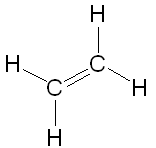# What is the ratio of carbon atoms to hydrogen atoms in the compound 2CH_2 rightleftharpoons...

## Question:

What is the ratio of carbon atoms to hydrogen atoms in the compound 2CH2 --> C2H4?

A. 1:9.

B. 9:1.

C. 3:6.

D. 6:3.

## Ratios

A ratio is used in mathematics, usually given in the format x:y, where x and y are numbers. It defines the relationship between two quantities. Reading a ratio we usually say we have y for every x.

This compound is called ethene and is shown below.There are 2 carbon atoms and 4 hydrogen atoms. For every 1 carbon atom, there are 2 hydrogen atoms. This gives us a ratio of 1:2 carbon to hydrogen atoms.

Going through the multiple-choice answers we need to find a ratio which equals 1:2. Answers A and B are obviously incorrect.

Answer C (3:6) when simplifying the ratio gives 1:2. This is therefore the correct answer.

Answer D (6:3) when simplifying the ratio gives 2:1 which is not the ratio we are looking for. This would be the ratio for hydrogen atoms to carbon atoms.